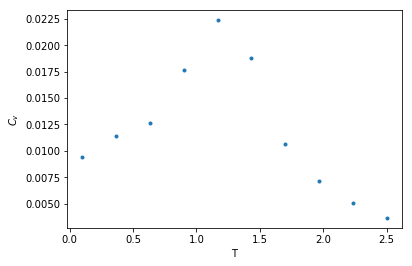# Monte Carlo Simulation of XY Model with Python

Published:

Python codes for XY model simulation.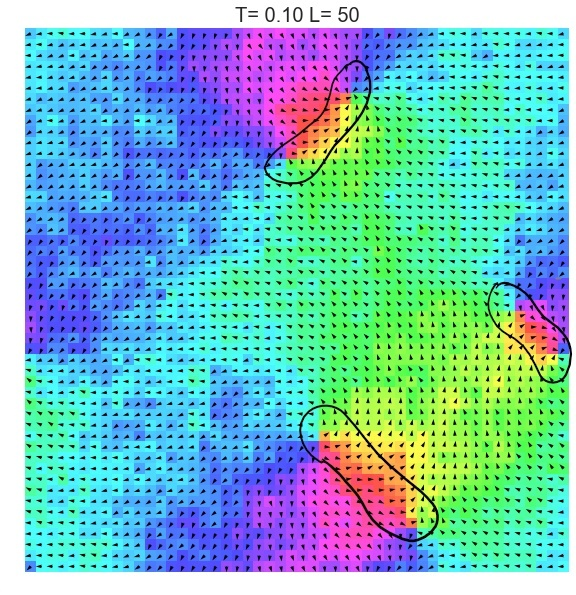## What’s it?

A python program used for Monte Carlo simulation (Metropolis algorithm) of XY model.

## What are the outputs?

• equilibrium spin configuration in differnet temperature
• thermal quantities of XY spin system in different temperature

## How to use this program

#### import the class

from XY_model import XYSystem


#### creating an object as a X-Y spin system with given width and temperature

Use XYSystem(temperature = , width = ) to creat a class object. Two variables can be assigned to initilize the system: the temperature and the width.

xy_system_1 = XYSystem(temperature  = 0.5, width = 15)


#### visulizingthe spin system

Using .show() to visulize the xy spin system as arrows on a two-dimensional plane.

xy_system_1.show()
print('Energy per spin:%.3f'%xy_system_1.energy)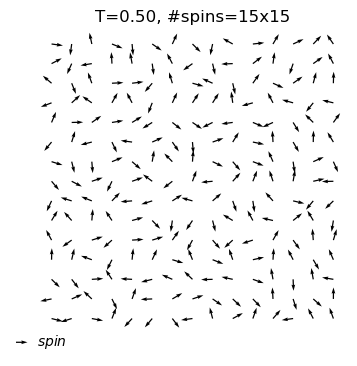Energy per spin:0.056


#### Now, let the system evolve to an equilibrium state

self.equilibrate(self,max_nsweeps=int(1e4),temperature=None,H=None,show = False) allows you to assign a new temperature, just simply do object.equilibrate(temperature = 3). If you want to keep the temperature defined before, leave it blank. And ·sohw=Ture will let show the configuration of the system for each 1000 sweeps.

xy_system_1.equilibrate(show=True)
xy_system_1.show()

#sweeps=1
energy=-0.60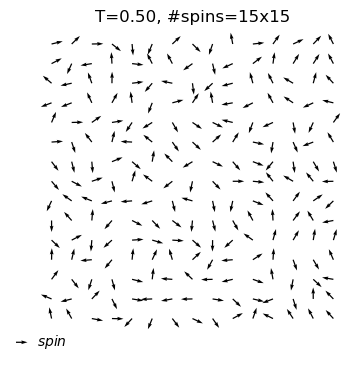equilibrium state is reached at T=0.5
#sweep=504
energy=-1.72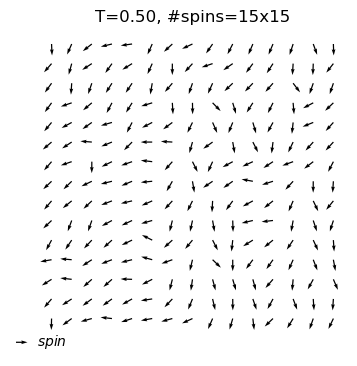#### Observing the thermal quantities in different temperature - annealing approach

xy_system_2 = XYSystem(width=7)
cool_dat=xy_system_2.annealing(T_init=2.5,T_final=0.1,nsteps = 10,show_equi=True)

equilibrium state is reached at T=2.5
#sweep=6802
energy=-0.48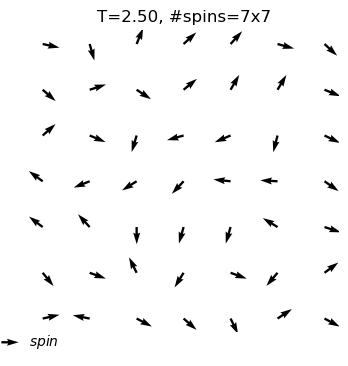equilibrium state is reached at T=2.2
#sweep=3429
energy=-0.48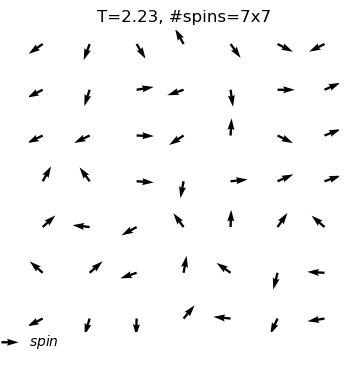equilibrium state is reached at T=2.0
#sweep=9999
energy=-0.47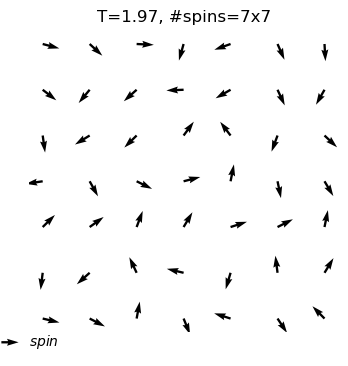equilibrium state is reached at T=1.7
#sweep=2809
energy=-0.60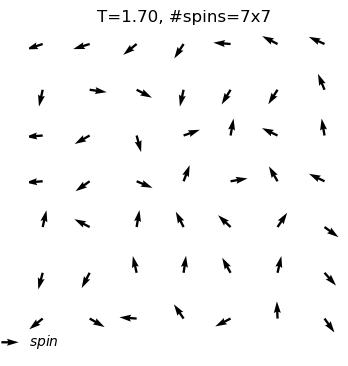equilibrium state is reached at T=1.4
#sweep=989
energy=-0.74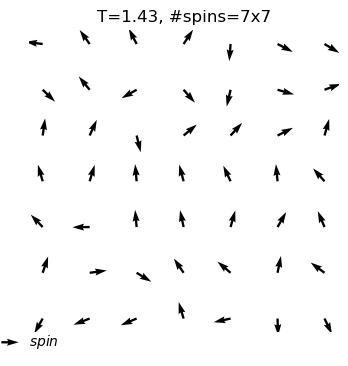equilibrium state is reached at T=1.2
#sweep=2253
energy=-1.35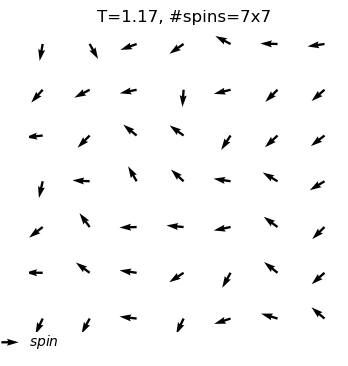equilibrium state is reached at T=0.9
#sweep=636
energy=-1.68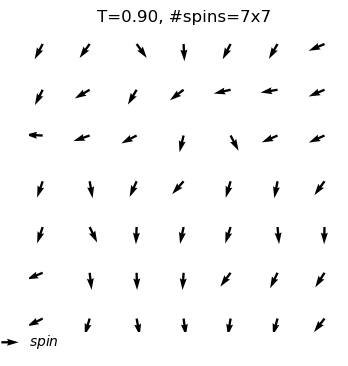equilibrium state is reached at T=0.6
#sweep=837
energy=-1.72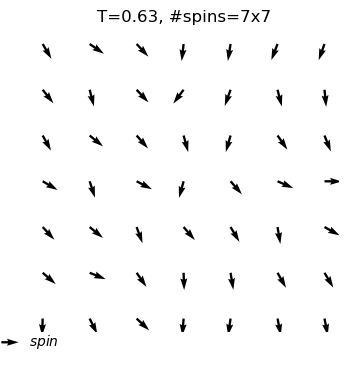equilibrium state is reached at T=0.4
#sweep=548
energy=-1.86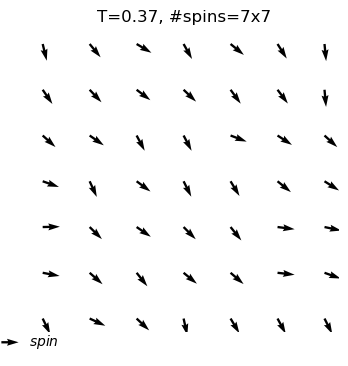equilibrium state is reached at T=0.1
#sweep=503
energy=-1.95
`